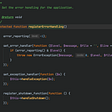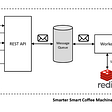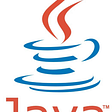# DRY vs. DAMP: the dichotomy

• For your production code, you should err on the side of the DRY principle
• For the test code, you should favor DAMP over DRY
`public class UserController{    public string Register(string userName, string email)    {        User userByName = _dbContext.Users            .SingleOrDefault(x => x.UserName == userName);        if (userByName != null)            return "User with such username already exists";        User userByEmail = _dbContext.Users            .SingleOrDefault(x => x.Email == email);        if (userByEmail != null)            return "User with such email already exists";        // Register the user        return "OK";    }    public string EditPersonalInfo(string userName, string email)    {        User currentUser = GetCurrentUser();        User userByName = _dbContext.Users            .SingleOrDefault(x =>                 x.UserName == userName &&                 x.UserName != currentUser.UserName);        if (userByName != null)            return "User with such username already exists";        User userByEmail = _dbContext.Users            .SingleOrDefault(x =>                 x.Email == email &&                 x.UserName != currentUser.Email);        if (userByEmail != null)            return "User with such email already exists";        // Update personal info        return "OK";    }}`
`public class UserController{    public string Register(string userName, string email)    {        if (UserNameAlreadyExists(userName, null))            return "User with such username already exists";        if (UserEmailAlreadyExists(email, null))            return "User with such email already exists";        // Register the user        return "OK";    }    public string EditPersonalInfo(string userName, string email)    {        User currentUser = GetCurrentUser();        if (UserNameAlreadyExists(userName, currentUser.UserName))            return "User with such username already exists";        if (UserEmailAlreadyExists(email, currentUser.Email))            return "User with such email already exists";        // Update personal info        return "OK";    }    private bool UserNameAlreadyExists(        string name, string currentUserName)    {        IQueryable<User> query = _dbContext.Users            .Where(x => x.UserName == name);        if (currentUserName != null)        {            query = query.Where(x => x.UserName != currentUserName);        }        User user = query.SingleOrDefault();        return user != null;    }    private bool UserEmailAlreadyExists(        string email, string currentUserEmail)    {        /* Same for email */    }}`
`[Fact]public void Division_by_zero(){    // Arrange    int dividend = 10;    int divisor = 0;    var calculator = new CalculatorController();    // Act    Envelope<int> response = calculator.Divide(dividend, divisor);    // Assert    response.IsError.Should().BeTrue();    response.ErrorCode.Should().Be("division.by.zero");}[Fact]public void Division_of_two_numbers(){    // Arrange    int dividend = 10;    int divisor = 2;    var calculator = new CalculatorController();    // Act    Envelope<int> response = calculator.Divide(dividend, divisor);    // Assert    response.IsError.Should().BeFalse();    response.Result.Should().Be(5);}`
`/* The initialization code that is common for both tests */int _dividend = 10;CalculatorController _calculator = new CalculatorController();[Fact]public void Division_by_zero(){    // Arrange    int divisor = 0;    // Act    Envelope<int> response = _calculator.Divide(_dividend, divisor);    // Assert    response.IsError.Should().BeTrue();    response.ErrorCode.Should().Be("division.by.zero");}[Fact]public void Division_of_two_values(){    // Arrange    int divisor = 2;    // Act    Envelope<int> response = _calculator.Divide(_dividend, divisor);    // Assert    response.IsError.Should().BeFalse();    response.Result.Should().Be(5);}`
• It introduces high coupling between tests — If you need to modify the calculator setup in one test, it will affect the other test too.
• It diminishes test readability — After extracting the two lines, you no longer see the full picture just by looking at individual tests. You have to examine the whole class in order to understand what the test does.

# The DRY vs. DAMP dichotomy is false

• For the production code, prefer DRY over DAMP
• For the test code, do the opposite choice.
`public static string GetStatus(bool isLocked){    return (isLocked ? "L" : "Unl") + "ocked";}`
`public static string GetStatus(bool isLocked){    return isLocked ? "Locked" : "Unlocked";}`
`/* The initialization code that is common for both tests */int _dividend = 10;CalculatorController _calculator = new CalculatorController();[Fact]public void Division_by_zero(){    // Arrange    int divisor = 0;    // Act    Envelope<int> response = _calculator.Divide(_dividend, divisor);    // Assert    response.IsError.Should().BeTrue();    response.ErrorCode.Should().Be("division.by.zero");}[Fact]public void Division_of_two_values(){    // Arrange    int divisor = 2;    // Act    Envelope<int> response = _calculator.Divide(_dividend, divisor);    // Assert    response.IsError.Should().BeFalse();    response.Result.Should().Be(5);}`

# DRY and DAMP as How-to’s and What-to’s

• The scenarios that need to be performed to verify the application’s correctness
• The steps that should be in those scenarios
`[Fact]public void Division_by_zero()[Fact]public void Division_of_two_numbers()`
`int dividend = 10;int divisor = 2;response.Result.Should().Be(5);`
• The what-to’s answer the question of what we are testing. They describe a test scenario using specific steps relevant for that scenario.
• The how-to’s contain the knowledge of how to implement those specific steps — how these are steps executed.
`public class CalculatorController{    private int _memorizedDividend;    public void Memorize(int dividend)    {        _memorizedDividend = dividend;    }    public Envelope<int> Divide(int divisor)    {        if (divisor == 0)           return Envelope<int>.Error(Errors.DivisionByZero);        int result = _memorizedDividend / divisor;        return Envelope<int>.Ok(result);    }}`
`[Fact]public void Division_by_zero_with_memorization(){    int dividend = 10;    int divisor = 0;    var calculator = new CalculatorController();    calculator.Memorize(dividend); // the additional method call    Envelope<int> response = calculator.Divide(divisor);    response.IsError.Should().BeTrue();    response.ErrorCode.Should().Be("division.by.zero");}[Fact]public void Division_of_two_values_with_memorization(){    int dividend = 10;    int divisor = 2;    var calculator = new CalculatorController();    calculator.Memorize(dividend); // the additional method call    Envelope<int> response = calculator.Divide(divisor);    response.IsError.Should().BeFalse();    response.Result.Should().Be(5);}`
`[Fact]public void Division_by_zero_with_memorization(){    int divisor = 0;    CalculatorController calculator =         CreateCalculatorWithMemorizedDividend(10);    Envelope<int> response = calculator.Divide(divisor);    response.IsError.Should().BeTrue();    response.ErrorCode.Should().Be("division.by.zero");}[Fact]public void Division_of_two_values_with_memorization(){    int divisor = 2;    CalculatorController calculator =         CreateCalculatorWithMemorizedDividend(10);    Envelope<int> response = calculator.Divide(divisor);    response.IsError.Should().BeFalse();    response.Result.Should().Be(5);}private CalculatorController     CreateCalculatorWithMemorizedDividend(int dividend){    var calculator = new CalculatorController();    calculator.Memorize(dividend);    return calculator;}`
• We don’t need to duplicate the knowledge of how to create the calculator with the memorized dividend.
• At the same time, we don’t compromise on the test readability. Thanks to the descriptive name of this method, we don’t need to examine the internals of this step in order to understand the attributes of the created calculator. Because we are keeping all the steps intact, we have the full context of what is going on in the tests.
`public class UserController{    public string Register(string userName, string email)    {        if (UserNameAlreadyExists(userName, null))            return "User with such username already exists";        if (UserEmailAlreadyExists(email, null))            return "User with such email already exists";        // Register the user        return "OK";    }    public string EditPersonalInfo(string userName, string email)    {        User currentUser = GetCurrentUser();        if (UserNameAlreadyExists(userName, currentUser.UserName))            return "User with such username already exists";        if (UserEmailAlreadyExists(email, currentUser.Email))            return "User with such email already exists";        // Update personal info        return "OK";    }    private bool UserNameAlreadyExists(        string name, string currentUserName)    {        /* Look in the database if the user name exists */    }    private bool UserEmailAlreadyExists(        string email, string currentUserEmail)    {        /* Look in the database if the user email exists */    }}`

# Summary

• DRY stands for “Don’t Repeat Yourself” and requires that any piece of domain knowledge has a single representation in your code base.
• DAMP stands for “Descriptive and Meaningful Phrases” and promotes the readability of the code.
• People often put these two principles in opposition to each other saying that:
• The dichotomy between DRY and DAMP is false. Both principles are equally important in both the production and test code.
• Think of DAMP and DRY in terms of what-to’s versus how-to’s:
• What-to’s answer the question of what we are doing; they describe the use case (in the case of the production code) or a test scenario (in the case of the test code) using specific steps
• How-to’s contain the knowledge of how to implement those specific steps
• The DRY principle should be applied to the how-to’s, whereas the DAMP principle should be applied to the what-to’s.

# Subscribe

--

--

--

Author of https://amzn.to/2QXS2ch. Founder of https://enterprisecraftsmanship.com/

Love podcasts or audiobooks? Learn on the go with our new app.

## Highlights from Chrome Dev Summit 2018## Paged-Based Pagination with Azure CosmosDB## Reactive Programming: The Hitchhiker’s Guide to map operators## Secrets of Git — git gotchas, tips & tricks## Improved error handling in your Lumen API## Intro to knapsack problemAuthor of https://amzn.to/2QXS2ch. Founder of https://enterprisecraftsmanship.com/

## Decouple Long-running Tasks from HTTP Request Processing — Using In-Memory Message Broker## Anemic Models vs Rich Models in Domain-Driven Design## CQRS & Event Sourcing I : Introduction## Introduction to CQRS and Event Sourcing